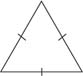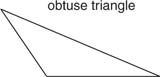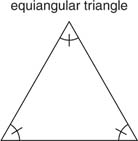## Classifying Triangles by Sides or Angles

Triangles can be classified either according to their sides or according to their angles. All of each may be of different or the same sizes; any two sides or angles may be of the same size; there may be one distinctive angle.

The types of triangles classified by their sides are the following:

• Equilateral triangle: A triangle with all three sides equal in measure. In Figure 1, the slash marks indicate equal measure.Figure 1 Equilateral triangle

• Isosceles triangle: A triangle in which at least two sides have equal measure (Figure 2).Figure 2 Isosceles triangles

• Scalene triangle: A triangle with all three sides of different measures (Figure 3).Figure 3 Scalene triangle

The types of triangles classified by their angles include the following:

• Right triangle: A triangle that has a right angle in its interior (Figure 4).Figure 4 Right triangle

• Obtuse triangle: A triangle having an obtuse angle (greater than 90° but less than 180°) in its interior. Figure 5 shows an obtuse triangle.Figure 5 Obtuse triangle

• Acute triangle: A triangle having all acute angles (less than 90°) in its interior (Figure 6).Figure 6 Acute triangle.

• Equiangular triangle: A triangle having all angles of equal measure (Figure 7).Figure 7 Equiangular triangleBecause the sum of all the angles of a triangle is 180°, the following theorem is easily shown.

Theorem 27: Each angle of an equiangular triangle has a measure of 60°.